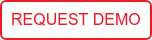# Blog

This is part 2 in a series discussing an approach to effective deployment of analytic models at scale.  You can find part 1 here.

Our first abstraction intended to aide the coordination of analytic designers and analytic deployment is the model.  As an abstract entity, a model has four main components.

• input (factors)
• output (scores and other data)
• state (including initial state, usually trained or fitted to historical data)
• the math (some times what is called the “scoring function”)

The math can be written or automatically generated in any language: Python, R, Java, etc.  The math is math, which by definition is language neutral.  Generally the structure of the math, for example GBMs or Random forests are templated in language and the specific structures and numeric values are filled in by a “fitting tool” like R or Spark or even by hand by the data scientist.  The math is like a black box, it takes the input (sometimes called factors), current state and generates the output (sometimes called y-hat or score or prediction or outcome or insight) and potentially some meta data.  This output data may then be passed to another stage in an analytics chain or used to monitor and report on the process etc.

We call the application of the input “scoring” but that’s just a technical jargon for taking a given input and a “current state” and applying the “action” of the math to produce an “output” for that particular input and state combination.

So let’s look two implementations of a trained neural net model of that fit this abstraction. One is in articulated in Python and one in R.  First let’s look at the Python Model:

```# input: array-double
# output: double```

# A Neural Net model
# y = f(W_2 f( W_1 x + b1) + b2) where
# x = input vector, y = output vector
# f is the activator function, and W_1, W_2 are the weights
# and b1, b2 are the bias vectors
# In this example, the neural net computes XOR of the inputs

import numpy as np
import math

# The Initial state

def begin():
global W_1, W_2, b_1, b_2, f
f = np.vectorize(activator)
W_1 = [[-6.0, -8.0], [-25.0, -30.0]]
b_1 = [4.0, 50.0]
W_2 = [[-12.0, 30.0]]
b_2 = -25.0

# The math with datum as type “input” and output at “yield”
def action(datum):
x = np.array(datum)
y = f(np.dot(W_2, f(np.dot(W_1, x) + b_1 ) ) + b_2)
yield y # the dot product returns a numpy array with one element

# Supporting functions
# Here we use a sigmoid logistic activator function
# but you can define your own
def activator(x):
return 1 / (1 + math.exp(-x))

And here is the R model:

```# input: array-double
# output: double```

# A Neural Net model
# y = f(W_2 f( W_1 x + b1) + b2) where
# x = input vector, y = output vector
# f is the activator function, and W_1, W_2 are the weights
# and b1, b2 are the bias vectors
# In this example, the neural net computes XOR of the inputs

# The Initial state

begin <- function(){
W_1 <<- matrix(c(-6, -8, -25, -30), nc = 2, byrow = T)
W_2 <<- matrix(c(-12, 30), nc = 1)
b_1 <<- c(4, 50)
b_2 <<- -25
}

# Supporting functions

activator <- function(x){
y <- 1/(1+exp(-x))
return(y)
}

# The math with datum as type “input” and output at “emit”

action <- function(datum){
x <- matrix(datum, nc = 1)
y <- activator(t(W_2) %*% activator((W_1 %*% x) + b_1) + b_2)
emit(y[])
}

Input “schema” or “type system” can be described with a language neutral system like Avro Schema:

`{"type" : "array", "items" : "double"}`

The output typing can be similarly described:

`{"type" : "double"}`

In fact, requiring a typed input / output set is essential to achieve scalable data science competency at scale.  You can also notice the regularity of structure and code points:

begin for the initial state

action(datum) where the math is defined over datum which is defined with the “input schema” describing an array of type double.  And some supporting functions for code organization, in this case the activator function of the neural net.

It turns out most analytic models can be easily conformed to this structure.  Moreover, doing so allows them to be executed by an agnostic engine which abstracts some of the messy DevOps and Data Engineering details away from this fairly clean math.  We’ll deal with the abstraction “streams” in our next blog.

By Stu Bailey